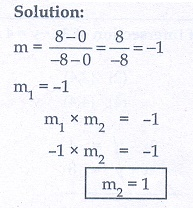Home | | Maths 10th Std | Multiple choice questions

# Multiple choice questions

Mathematics : Coordinate Geometry: Multiple choice questions with answers / choose the correct answer with answers - Maths Book back 1 mark questions and answers with solution for Exercise Problems

Multiple choice questions

1. The area of triangle formed by the points (−5,0) , (0,−5) and (5,0) is

(1) 0 sq.units

(2) 25 sq.units

(3) 5 sq.units

(4) none of these2. A man walks near a wall, such that the distance between him and the wall is 10 units. Consider the wall to be the Y axis. The path travelled by the man is

(1) = 10

(2) = 10

(3) = 0

(4) = 03. The straight line given by the equation x = 11 is

(1) parallel to X axis

(2) parallel to Y axis

(3) passing through the origin

(4) passing through the point (0,11)4. If (5,7), (3,p) and (6,6) are collinear, then the value of p is

(1) 3

(2) 6

(3) 9

(4) 125. The point of intersection of 3x − y = 4 and x + y = 8 is

(1) (5,3)

(2) (2,4)

(3) (3,5)

(4) (4,4)6. The slope of the line joining (12, 3) , (4,a) is 1/8. The value of ‘a’ is

(1) 1

(2) 4

(3) -5

(4) 27. The slope of the line which is perpendicular to a line joining the points (0,0) and (–8,8) is

(1) -1

(2) 1

(3) 1/3

(4) -88. If slope of the line PQ is 1/√3 then slope of the perpendicular bisector of PQ is

(1) √3

(2) -√3

(3) 1/√3

(4) 09. If A is a point on the Y axis whose ordinate is 8 and B is a point on the X axis whose abscissae is 5 then the equation of the line AB is

(1) 8x + 5y = 40

(2) 8x − 5y = 40

(3) = 8

(4) y = 510. The equation of a line passing through the origin and perpendicular to the line 7x − 3y + 4 = 0 is

(1) 7x − 3y + 4 = 0

(2) 3x − 7y + 4 = 0

(3) 3x + 7y = 0

(4) 7x − 3y = 011. Consider four straight lines

(i) l1 : 3y = 4x + 5

(ii) l 2  : 4y = 3x −1

(iii) l3: 4y + 3x = 7

(iv) l 4  : 4x + 3y = 2

Which of the following statement is true ?

(1) l1 and  l2 are perpendicular

(2) l1 and l4 are parallel

(3) land l4 are perpendicular

(4) l2 and   l3 are parallel12. A straight line has equation 8y = 4x + 21 . Which of the following is true

(1) The slope is 0.5 and the y intercept is 2.6

(2) The slope is 5 and the y intercept is 1.6

(3) The slope is 0.5 and the y intercept is 1.6

(4) The slope is 5 and the y intercept is 2.613. When proving that a quadrilateral is a trapezium, it is necessary to show

(1) Two sides are parallel.

(2) Two parallel and two non-parallel sides.

(3) Opposite sides are parallel.

(4) All sides are of equal length.14. When proving that a quadrilateral is a parallelogram by using slopes you must find

(1) The slopes of two sides

(2) The slopes of two pair of opposite sides

(3) The lengths of all sides

(4) Both the lengths and slopes of two sides15. (2, 1) is the point of intersection of two lines.

(1)  y  3 = 0;  3 7 = 0

(2) + y = 3; 3x + y = 7

(3) 3x + y = 3;  x + y = 7

(4) + 3 3 = 0;  x −y − 7 = 0